# sklearn.datasets.load_diabetes¶

Load and return the diabetes dataset (regression).

 Samples total 442 Dimensionality 10 Features real, -.2 < x < .2 Targets integer 25 - 346

Note

The meaning of each feature (i.e. feature_names) might be unclear (especially for ltg) as the documentation of the original dataset is not explicit. We provide information that seems correct in regard with the scientific literature in this field of research.

Read more in the User Guide.

Parameters:
return_X_ybool, default=False

If True, returns (data, target) instead of a Bunch object. See below for more information about the data and target object.

New in version 0.18.

as_framebool, default=False

If True, the data is a pandas DataFrame including columns with appropriate dtypes (numeric). The target is a pandas DataFrame or Series depending on the number of target columns. If return_X_y is True, then (data, target) will be pandas DataFrames or Series as described below.

New in version 0.23.

scaledbool, default=True

If True, the feature variables are mean centered and scaled by the standard deviation times the square root of n_samples. If False, raw data is returned for the feature variables.

New in version 1.1.

Returns:
dataBunch

Dictionary-like object, with the following attributes.

data{ndarray, dataframe} of shape (442, 10)

The data matrix. If as_frame=True, data will be a pandas DataFrame.

target: {ndarray, Series} of shape (442,)

The regression target. If as_frame=True, target will be a pandas Series.

feature_names: list

The names of the dataset columns.

frame: DataFrame of shape (442, 11)

Only present when as_frame=True. DataFrame with data and target.

New in version 0.23.

DESCR: str

The full description of the dataset.

data_filename: str

The path to the location of the data.

target_filename: str

The path to the location of the target.

(data, target)tuple if return_X_y is True

Returns a tuple of two ndarray of shape (n_samples, n_features) A 2D array with each row representing one sample and each column representing the features and/or target of a given sample.

New in version 0.18.

## Examples using sklearn.datasets.load_diabetes¶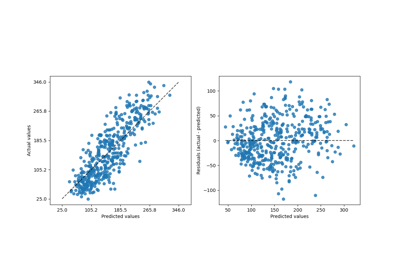Release Highlights for scikit-learn 1.2

Release Highlights for scikit-learn 1.2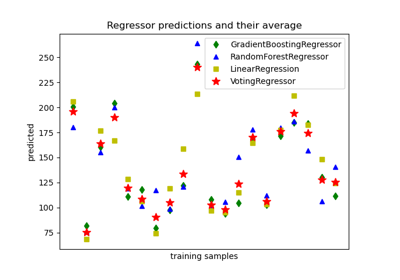Plot individual and voting regression predictions

Plot individual and voting regression predictions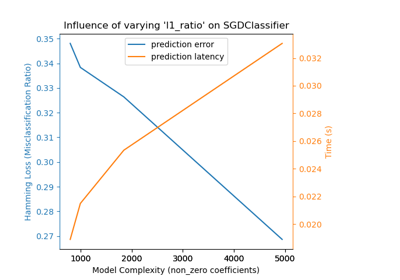Model Complexity Influence

Model Complexity Influence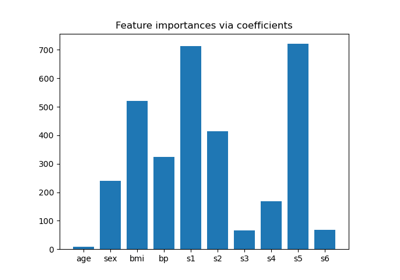Model-based and sequential feature selection

Model-based and sequential feature selectionLasso and Elastic Net

Lasso and Elastic Net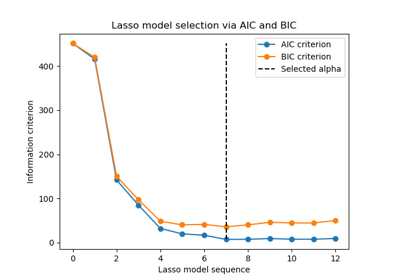Lasso model selection via information criteria

Lasso model selection via information criteria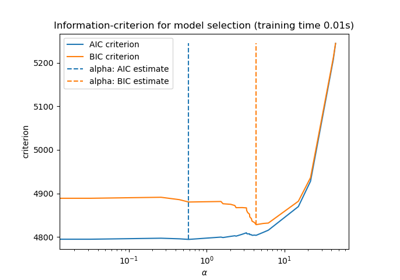Lasso model selection: AIC-BIC / cross-validation

Lasso model selection: AIC-BIC / cross-validationLasso path using LARS

Lasso path using LARSLinear Regression Example

Linear Regression ExampleSparsity Example: Fitting only features 1 and 2

Sparsity Example: Fitting only features 1 and 2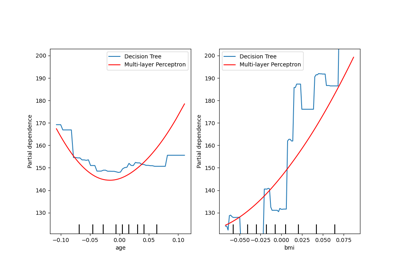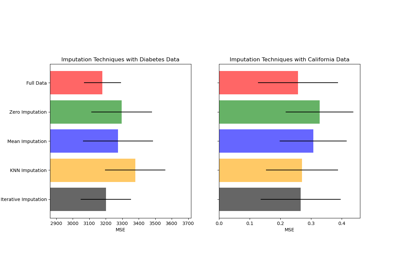Imputing missing values before building an estimator

Imputing missing values before building an estimator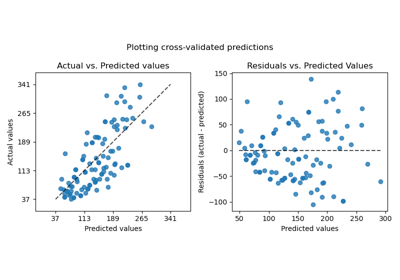Plotting Cross-Validated Predictions

Plotting Cross-Validated PredictionsCross-validation on diabetes Dataset Exercise

Cross-validation on diabetes Dataset Exercise